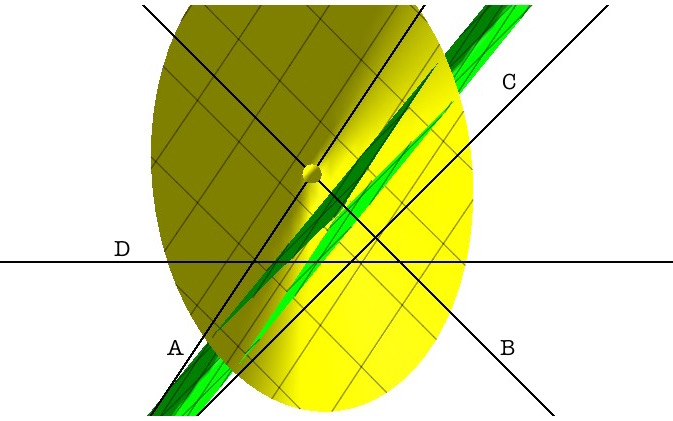THE CONIC GEOMETRY OF RECTANGLES INSCRIBED IN LINES
BRUCE OLBERDING AND ELAINE A. WALKER
Abstract. We develop a circle of ideas involving pairs of lines in the plane, intersections of hyperbolically rotated elliptical cones and the locus of the centers of rectangles inscribed in lines in the plane. (Read the pdf on the arXiv.)

This page contains supplementary graphics for our article, The Conic Geometry of Rectangles Inscribed in Lines. All of the graphs were generated in MapleTM.

The first two graphs show an intersection of two HR-cones. The red curve in the first graph is the intersection of the two cones. In the 2nd graph the cones have been removed to show the space curve that is the intersection of the two cones.This video shows different views of the space curve in the above two graphs. At 2 seconds, it is clear that the upper left branch does not lie in a plane. (See Remark 4.12.) The video ends with a view directly from above the cones, which shows the projection of the space curve to the xy-plane. This hyperbola in the plane is the rectangle locus for the two pairs of lines (not shown) that are comprised of the generating lines for the two cones.
The following graph shows a level curve at height 1 of an HR cone and the generating lines of the HR cone. Intersecting with the unit circle locates the cone lines as in Remark 3.7.The following series of examples illustrates the rectangle loci for different pairings of four lines A, B, C, D. The rectangle loci are found as in Lemma 4.3 by intersecting the HR-cones generated by the different pairings. For example, the first snapshot involves the intersection of the HR-cones generated by the pairs AB and CD. The snapshot is taken from above, looking directly down into the two HR-cones. (The two cones are only shown below a fixed height.) The four lines are superimposed on the cross-section in order that their relationship to the two cones is more apparent. The small spheres mark the center of the cone of corresponding color as well as the paired lines that determine the cone. By Lemma 4.3, the projection to the xy-plane of the intersection of the cones is the rectangle locus for the two pairs. In the first three snapshots, this projection is a non-degenerate hyperbola.
AB, CDAC, BDAB, ACAB, BCAB, BDAC, BCAC, DC !

coming soon..